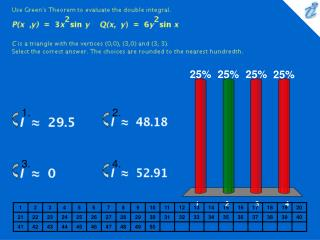# {image} {image} {image} {image} - PowerPoint PPT PresentationDownload Presentation{image} {image} {image} {image}

{image} {image} {image} {image}Download Presentation## {image} {image} {image} {image}

- - - - - - - - - - - - - - - - - - - - - - - - - - - E N D - - - - - - - - - - - - - - - - - - - - - - - - - - -
##### Presentation Transcript

1. 1. 2. 3. 4. Use Green's Theorem to evaluate the double integral. {image} C is a triangle with the vertices (0,0), (3,0) and (3, 3). Select the correct answer. The choices are rounded to the nearest hundredth. • {image} • {image} • {image} • {image}

2. Use Green's Theorem to evaluate the line integral along the given positively oriented curve. {image} and C is the boundary of the region enclosed by the parabola {image} and the line y = 16. • 8,192 • -3,968 • -7,936

3. 1. 2. 3. 4. Use Green's Theorem to evaluate the line integral along the given positively oriented curve. {image} and C is the boundary of the region between the circles {image} . Choose the correct answer. • {image} • {image} • {image} • {image}

4. 1. 2. 3. 4. A particle starts at the point (-2, 0),moves along the x - axis to (2, 0) and then along the semicircle {image} to the starting point. Use Green's Theorem to find the work done on this particle by the force field {image} Choose the correct answer. • {image} • {image} • {image} • 0